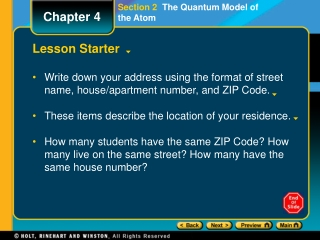DownloadDownload PresentationSection 2 The Quantum Model of the Atom

# Section 2 The Quantum Model of the Atom

Download Presentation## Section 2 The Quantum Model of the Atom

- - - - - - - - - - - - - - - - - - - - - - - - - - - E N D - - - - - - - - - - - - - - - - - - - - - - - - - - -
##### Presentation Transcript

1. Section 2 The Quantum Model of the Atom Chapter 4 Lesson Starter • Write down your address using the format of street name, house/apartment number, and ZIP Code. • These items describe the location of your residence. • How many students have the same ZIP Code? How many live on the same street? How many have the same house number?

2. Section 2 The Quantum Model of the Atom Chapter 4 Lesson Starter, continued • In the same way that no two houses have the same address, no two electrons in an atom have the same set of four quantum numbers. • In this section, you will learn how to use the quantum-number code to describe the properties of electrons in atoms.

3. Section 2 The Quantum Model of the Atom Chapter 4 Objectives • Discuss Louis de Broglie’s role in the development of the quantum model of the atom. • Compare and contrast the Bohr model and the quantum model of the atom. • Explain how the Heisenberg uncertainty principle and the Schrödinger wave equation led to the idea of atomic orbitals.

4. Section 2 The Quantum Model of the Atom Chapter 4 Objectives, continued • List the four quantum numbers and describe their significance. • Relate the number of sublevels corresponding to each of an atom’s main energy levels, the number of orbitals per sublevel, and the number of orbitals per main energy level.

5. Section 2 The Quantum Model of the Atom Chapter 4 Electrons as Waves • French scientist Louis de Broglie suggested that electrons be considered waves confined to the space around an atomic nucleus. • It followed that the electron waves could exist only at specific frequencies. • According to the relationship E = hν, these frequencies corresponded to specific energies—the quantized energies of Bohr’s orbits.

6. Section 2 The Quantum Model of the Atom Chapter 4 Electrons as Waves, continued • Electrons, like light waves, can be bent, or diffracted. • Diffraction refers to the bending of a wave as it passes by the edge of an object or through a small opening. • Electron beams, like waves, can interfere with each other. • Interference occurs when waves overlap.

7. Section 2 The Quantum Model of the Atom Chapter 4 The Heisenberg Uncertainty Principle • German physicist Werner Heisenberg proposed that any attempt to locate a specific electron with a photon knocks the electron off its course. • The Heisenberg uncertainty principle states that it is impossible to determine simultaneously both the position and velocity of an electron or any other particle.

8. Section 2 The Quantum Model of the Atom Chapter 4 The Schrödinger Wave Equation • In 1926, Austrian physicist Erwin Schrödinger developed an equation that treated electrons in atoms as waves. • Together with the Heisenberg uncertainty principle, the Schrödinger wave equation laid the foundation for modern quantum theory. • Quantum theory describes mathematically the wave properties of electrons and other very small particles.

9. Section 2 The Quantum Model of the Atom Chapter 4 The Schrödinger Wave Equation, continued • Electrons do not travel around the nucleus in neat orbits, as Bohr had postulated. • Instead, they exist in certain regions called orbitals. • An orbital is a three-dimensional region around the nucleus that indicates the probable location of an electron.

10. Section 2 The Quantum Model of the Atom Chapter 4 Atomic Orbitals and Quantum Numbers • Quantum numbers specify the properties of atomic orbitals and the properties of electrons in orbitals. • The principal quantum number, symbolized by n, indicates the main energy level occupied by the electron. • The angular momentum quantum number, symbolized by l, indicates the shape of the orbital.

11. Section 2 The Quantum Model of the Atom Chapter 4 Atomic Orbitals and Quantum Numbers, continued • The magnetic quantum number, symbolized by m, indicates the orientation of an orbital around the nucleus. • The spin quantum number has only two possible values—(+1/2 , −1/2)—which indicate the two fundamental spin states of an electron in an orbital.

12. Section 2 The Quantum Model of the Atom Chapter 4 Shapes of s, p, and d Orbitals

13. Section 2 The Quantum Model of the Atom Chapter 4 Electrons Accommodated in Energy Levels and Sublevels

14. Section 2 The Quantum Model of the Atom Chapter 4 Electrons Accommodated in Energy Levels and Sublevels

15. Section 2 The Quantum Model of the Atom Chapter 4 Quantum Numbers of the First 30 Atomic Orbitals